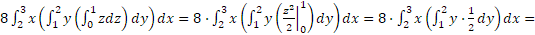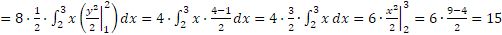## Hey! Need a Math Help?

#### Free Integral Calculator

If you need any help with algebra, calculus, online classes, courseworks, do my math homework statistics or any math related subject you can rely on us. Our professionals can help. Overnight delivery! 24/7 customer support!

## Integral Calculation

Integral calculus emerged when the scientists were searching how to compute the areas of surfaces, plane figures, solid body volumes, and addressed problems in hydrodynamics, statistics and other physics domains. An example of a problem that contributed to the development of integral calculus is finding the object’s law of motion along a line given that the velocity of this object is known.

Historically integral calculus evolved as a domain of mathematical analysis, which developed as a result of solving two key problems: finding the function by its derivative, and determining the area bounded by a certain graph or three-dimensional surface(s) at a certain interval(s). The first problem stimulated the evolution of the analytical meaning of an integral (indefinite integral), and the second problem gave birth to the concept of a definite integral.

Integrals are now widely used in different domains of research and computing. Integral calculus can be used to find the average value of a function within a given interval, to compute the areas between curves, to determine the volumes of rotating objects or regions, etc. In addition, integral calculus is widely applied in physics: it can be used to determine the amounts of work needed to move or rotate an object, to address kinematics problems, to study and model the motion and interaction of objects, to find center of mass, to assess the probability of certain events. Thus, understanding of integral calculus and the ability to work with different integral types is critically important in many domains.

## Types of Integrals

There are two major classes of integrals: indefinite and definite. An indefinite integral denotes a function, the derivative of which yields a given function. Indefinite integrals do not have limits of integration. In other words, indefinite integration is an opposite operation to analytical differentiation. The equation for an indefinite integral can be written as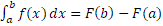. This equation reads as follows: the indefinite integral of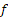with respect to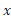.

A definite integral has limits of integration. In its basic form and in the context of a two-dimensional function, a definite integral equals to the area under the function’s curve within a given interval. Riemann, one of the founders of integral calculus, described the definite integral as the result of a limiting procedure approximating an area between two curves by breaking the area into vertical thin slabs. The equation for an definite integral can be written as. This equation reads as follows: the definite integral offrom a to b with respect to.

There are many types of definite integrals differing by the boundness of the integral sum (proper and improper integrals) and by the number of variables in the function (double, triple and multiple integrals). All types of integrals described above will be discussed in more details and with examples in the next sections.

## Antiderivatives

The antiderivative is an important concept in integration. Antiderivative of a function gets back to the original function; in other words, if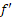is the derivative of a function, thenis an antiderivative for. For example, the derivative ofequals to, and thereforeis an antiderivative for.

Precise definition of an antiderivative is the following: functionis an antiderivative of functionon the intervaliffor all. The concept of antiderivative is used for calculating various types of integrals. It is important to note that since the derivative of a constant equals to 0, the antiderivative actually represents a family of antiderivatives differing by a constant C.

## Indefinite integrals

The indefinite integral of a given function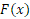is written down as, and the relationship between these functions is represented by the following equation:describes the function that is being integrated (the integrand),is the antiderivative,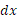is the integrating agent, and C is the integration constant.

Each functionhas an indefinite number of integrals which differ by the constant C. Indeed, since the derivative of a constant is 0, this means that integrating a function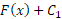and integrating a function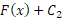will yield the same result,.

Example of calculating an indefinite integral: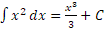. The result can be verified using derivatives:. Indeed, the derivative of the function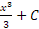equals to initial function,, which proves that the calculation is correct.

## Limit of sum

One of the problems solved by integration is finding the area under the curve within a certain interval. To address this problem, the concept of Riemann sums was coined. Let us assume the goal is to determine the area under the function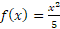bounded by the lines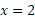and. The figure below demonstrates the target area that should be calculated.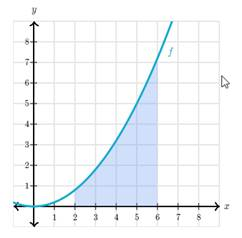The area under the curve can be approximated by breaking the x-interval betweenandinto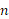intervals of equal width, and computing the sum of rectangles, which intersect with the function as displayed on the figure below.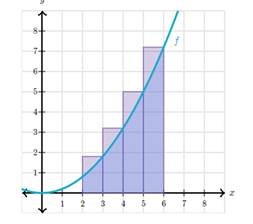This approximation of the area can be written as: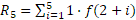. To get a more precise estimation of the area under the curve, it is reasonable to increase, therefore reducing the size of intervals.

In the general form, this approximation of area under the curve is expressed using the following formula: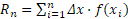, where n is the number of intervals, a is the start of the interval, and b is the end of the interval,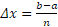, and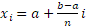. This sum is referred to as Riemann sum. As the value of n increases, the approximation of area under the curve becomes better. Assuming that n increases to infinity, it is possible to define the area under the curve as the limit of Riemann sum:.

## Definite integrals

The limit of Riemann sum is called definite integral. It is denoted as follows. The definite integral of a continuous function f over the interval [a, b]equals to the limit of Riemann sum as n approaches infinity.The value of definite integral can be computed using antiderivative: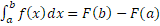, where https://essay.ws/wp-content/uploads/2019/05/fx.png is the antiderivative of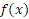with constant C = 0.
Example: let us calculate the area under the curveover the interval [2, 6]. This area is computed using the following definite integral: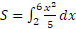. The antiderivative for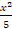is: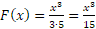. Therefore,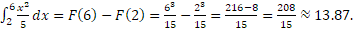## Definite integrals

The limit of Riemann sum is called definite integral. It is denoted as follows. The definite integral of a continuous function f over the interval [a, b]equals to the limit of Riemann sum as n approaches infinity.The value of definite integral can be computed using antiderivative:, where https://essay.ws/wp-content/uploads/2019/05/fx.png is the antiderivative ofwith constant C = 0.
Example: let us calculate the area under the curveover the interval [2, 6]. This area is computed using the following definite integral:. The antiderivative foris:. Therefore,## Proper integrals

The definite integral is defined for a functionover the interval [2,6] with the assumptions that the interval is finite, and thatis continuous over this interval. If both of these assumptions are satisfied, such integral is referred to as proper integral, and is computed according to the definite integral rules: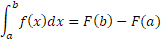Example of calculating proper integral: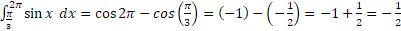## Improper integrals

In the previous section, the concept definite integral was based on two key assumptions: finiteness of the integration interval and the continuity of the integrated function over this interval. Such integrals are also called proper integrals. If either of these conditions is violated, then the integral is referred to as improper.

One example of an improper integral is the case when one of the integration limits is infinite: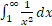. Another example of an improper integral is the case when the function has at least one infinite discontinuity over the interval: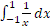(the functionbecomes infinite when x approaches 0 from both sides).

Improper integral with the right side of the interval being infinite can be determined as: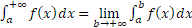If such limit exists, then the improper integral converges; otherwise, it diverges. The same approach is used to compute the improper integral with the left side of the integral being infinite: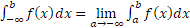An improper integral calculated on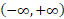is rewritten as a sum of two integrals, one with infinite lower boundary, and one with infinite upper boundary: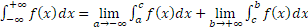, whereis any convenient number where the function is defined and continuous. The same approach is used for integrals that contain discontinuities over the interval: the integral is represented as the sum of two integrals with the point of discontinuity separating the original integration interval into two intervals.
Example of calculating an improper integral: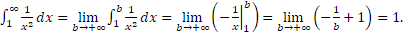The given improper integral converges to 1.

## Multiple Integrals

Unlike a single integral which is determined for a given function over an interval, a multiple integral is determined for a function with n variables: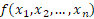over a domain D bounded in an n-dimensional space. In the general form, such integrals can be written as: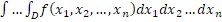.
Multiple integrals are frequently used in engineering and physics for computing volumes, masses, centers of masses, etc. The most frequently used multiple integrals are double and triple integrals. Definitions and examples of these integrals are presented in the next sections.

## Double Integrals

A single definite integral is denoted as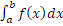. While single integrals operate over an interval, double integrals operate over the plane (two dimensions). A double integral is written as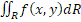, where f(x,y) is the integrated function defined over the plane, and R is the region of integration on the (x,y) plane. The single definite integral calculates the area under the curve over a defined interval, and double integral calculates the volume under the surface defined by z=f(x,y) over the region R.

Double integrals are computed sequentially: it is necessary to work out the limits of integration for x and y, then to perform integration for y variable (assuming x is constant), and to integrate the result for x variable. Assuming thatand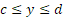, the process of calculating a double integral is represented by the following equation: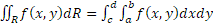Example of calculating a double integral: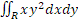, where R is a rectangle defined by the following boundaries: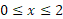and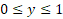.

This integral can be calculated as follows: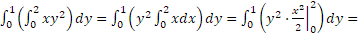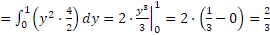## Triple integrals

Triple integrals represent a concept similar to double integrals, but the area of integration in this case is not an area but rather a three-dimensional surface. A triple integral can be represented as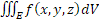, where f(x,y,z) is the integrated function defined over the three-dimensional shape E, and E is the region of integration in the (x,y,z) three-dimensional space. Such integrals are used to compute the volumes and densities of three-dimensional figures as well as moments, centers of mass and inertia.

Triple integrals are also calculated sequentially, similarly to double integrals: the integration is performed by one dimension, assuming that other dimensions are constant, then by the second dimension, and finally by the third dimension. Example of calculating a triple integral: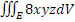, where E is defined by the following boundaries: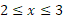, and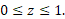It can be written as: